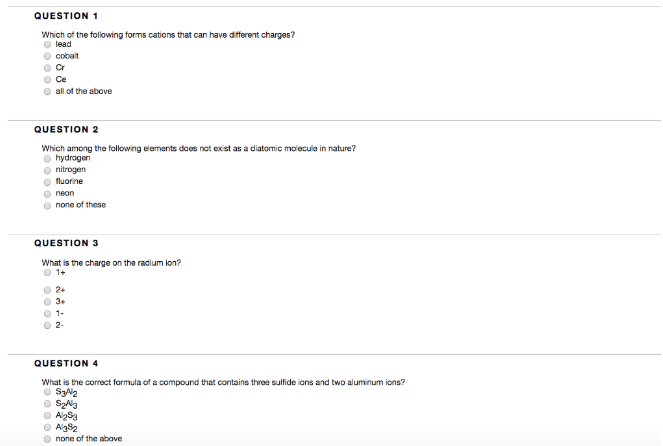# Problem: Which of the following forms cations that can have different charges? a. lead b. cobalt c. Cr d. Ce e. all of the aboveWhich among the following elements does not exist as a diatomic molecule in nature? a. hydrogen b. nitrogen c. fluorine d. neon e. none of these What is the charge on the radium ion? a. 1+ b. 2+ c. 3+ d. 1- e. 2-What is the correct formula of a compound that contains three sulfide ions and two aluminum ions? a. S3Al2 b. S2Al3 c. Al2S3 d. Al3S2 e. none of the above

###### FREE Expert Solution###### Problem Details

Which of the following forms cations that can have different charges?

b. cobalt

c. Cr

d. Ce

e. all of the above

Which among the following elements does not exist as a diatomic molecule in nature?

a. hydrogen

b. nitrogen

c. fluorine

d. neon

e. none of these

What is the charge on the radium ion?

a. 1+

b. 2+

c. 3+

d. 1-

e. 2-

What is the correct formula of a compound that contains three sulfide ions and two aluminum ions?

a. S3Al2

b. S2Al3

c. Al2S3

d. Al3S2

e. none of the above